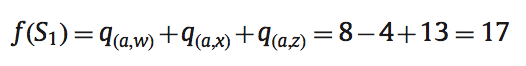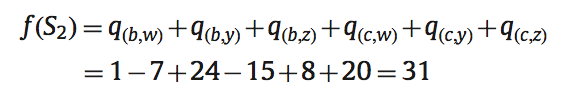# Bipartite unconstrained 0-1 Quadratic Programming Problem

A. Duarte, M. Laguna, R. Martí, Jesús Sánchez-Oro (2015)
Optsicom project, University of Valencia (Spain)

# Problem Description

The bipartite unconstrained 0-1 quadratic programming pro- blem (BQP) consists of selecting a subgraph that maximizes the sum of the weights associated with the chosen vertices and the edges that connect them. The problem is defined on a complete bipartite graph G = (V,E), with I = {1, 2, ..., m} representing the set of vertices in the left-hand side of the graph, J = {1, 2, ..., n} representing the set of vertices in the right-hand side of the graph, E representing the set of edges that connect the vertices in I with the vertices in J, and V = I ∪ J. There is a weight cv associated with each vertex v ∈ V . There is also a weight qij that corresponds to the edge connecting vertices i ∈ I and j ∈ J. The problem consists of selecting S ⊆ V such that the following function is maximizedFig.1 shows an example with I={a,b,c}, J={x,y,z} and the table of edge weights. We assume that all vertex weights are zeroWe point out that weights, either on the vertices or the edges, can be positive, negative or zero. Consider a solution S1={a,w,x,z}. The objective function value of this solution isA better solution is obtained by making the following vertex selections: S2 = {b,c,w,y,z}. The objective function value of solution S2 isIn this case, an increase in the number of vertices from solution S1 to solution S2 resulted in an increase in the objective function of 14 units (31–17=14). However, this is not necessarily true in all cases. For instance, selecting a and c on the left side and y and z on the right side results in an objective function value of 38. This solution has four vertices and is better than solution S2 that has 5 vertices.

# State of the Art Methods

BQP has not been thoroughly studied from a heuristic point of view. To the best of our knowledge, there exist one article that describe several heuristics for this problem (Karapetyan and Punnen, 2013). This work develops 24 heuristics that are grouped into three families: fast-heuristics, slow-heuristics and row-merge heuristics.

# Instances (VSPLIB)

The data for the experiments were taken from the literature. More precisely, we employ the tested generated by Karapetyan and Punnen (2013). All the details on how the data instances were generated are found in Section 4 of Karapetyan and Punnen (2013). The testbed2 contains the following five graph types: random, max biclique, max induced subgraph, maxcut, and matrix factorization. For each graph type, we consider 17 instances for a total of 85. The 17 instances are divided into three groups


• 7 small instances (m = {20, 25, 30, 35, 40, 45, 50} and n = 50)
• 
• medium instances (m = {200, 400, 600, 800, 1000} and n = 1000)
• 
• large instances (m = {1000, 2000, 3000, 4000, 5000} and n = 5000)